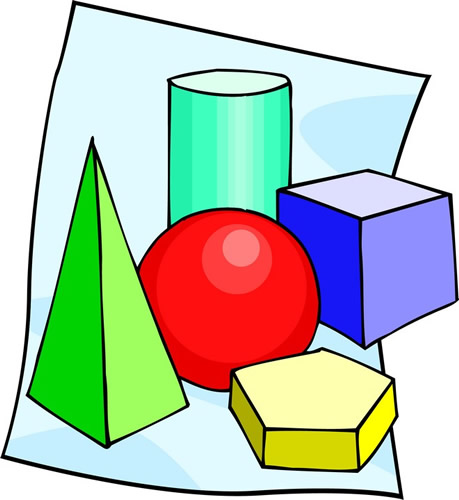• Welcome to the course page for Regents Geometry.  This course prepares students to be successful on the Common Core Geometry Regents Exam which will be given in June 2016.

Below is a an overview of the course and the topics covered.

Overview of Year

Geometry Mathematics Curriculum

 SEPT OCT NOV DEC JAN FEB MARCH APRIL MAY JUNE Unit 1 Shadows -  25 days Unit 2 Geometry By Design - 25 days Unit 3 Do Bees Build It Best? -5 days Unit 4 Orchard Hideout -25 days

 Unit 1 Understanding Essential Question Shadows ·         Similarity and congruence ·         Similarity and triangles ·         Similarity and right triangle trigonometry ·         Similarity and proportionality ·         Angle sum formulas for polygons ·         Triangle inequality and extension to other polygons ·         Angles relationships in parallel lines cut by a transversal ·         Vertical angles and angle sums in polygons ·         Solving problems using similarity ·         Solving problems using right triangle trigonometry ·         Similarity proofs ·         Radian measure What is the relationship between similarity and size transformations?   How can deductive arguments be used to show similarity of two figures?   What are the special properties of similar triangles and how do these properties lead to the definition of the trigonometric ratios? Performance Task:

 Unit 2 Understanding Essential Question Geometry by Design ·         Basic constructions ·         Triangle congruence theorems ·         Use of triangle congruence theorems to prove properties of triangles and quadrilaterals ·         Transformations ·         Use of transformations to prove properties of triangles and quadrilateral, ·         Triangle congruence proofs ·         Use of transformations to solve problems What is the relationship between congruence and the rigid transformations?   How can deductive arguments be used to show congruence of two figures?   What are the special properties of triangles and quadrilaterals? Performance Task:

 Unit 3 Understanding Essential Question Do Bees Build It Best? ·         Area and perimeter ·         Surface area and volume ·         Pythagorean theorem ·         Properties of trigonometric ratios in triangles ·         Mean proportional ·         Relationships between the perimeters, areas, surface areas, and volumes of similar figures How can area and volume formulas be developed?   How can area and volume formulas be used to solve problems? Performance Task:

 Unit 4 Understanding Essential Question Orchard Hideout ·         Coordinate geometry ·         Distance formula ·         Midpoint formula ·         Slope and parallel and perpendicular lines ·         Equation of a circle ·         Completing the square ·         Points equidistant from the endpoints of a segment are on the perpendicular bisector of the segment (and vice versa) ·         Coordinate proofs ·         Centers of triangles ·         Constructions ·         Circle theorems ·         Exterior angle theorem for triangles ·         Midpoint connector theorem ·         Area of sectors of circles ·         Volume formulas How can the coordinate plane be used to express geometric relationships algebraically?   How can coordinates be used to prove geometric properties? Performance Task: